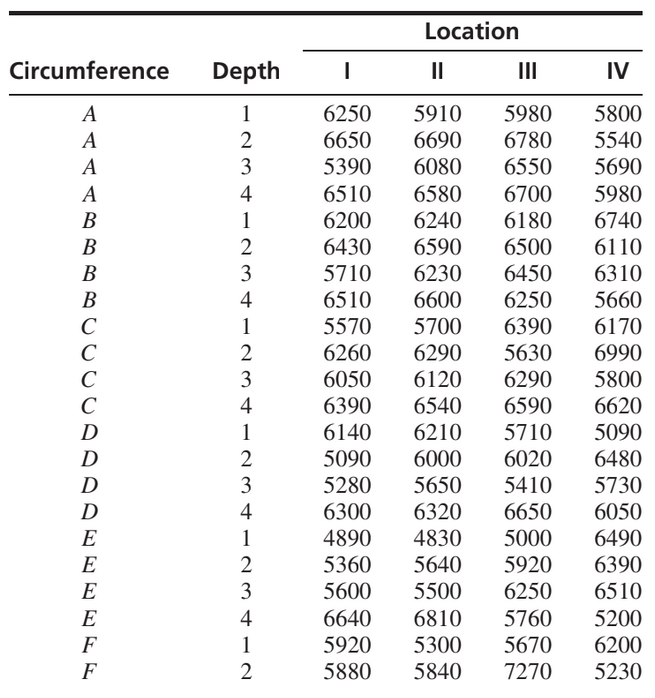×
Get Full Access to Statistics For Engineers And Scientists - 4 Edition - Chapter 9 - Problem 18se
Get Full Access to Statistics For Engineers And Scientists - 4 Edition - Chapter 9 - Problem 18se

×

# The Williamsburg Bridge is a suspension bridge that spansISBN: 9780073401331 38

## Solution for problem 18SE Chapter 9

Statistics for Engineers and Scientists | 4th Edition

• Textbook Solutions
• 2901 Step-by-step solutions solved by professors and subject experts
• Get 24/7 help from StudySoup virtual teaching assistantsStatistics for Engineers and Scientists | 4th Edition

4 5 1 413 Reviews
19
2
Problem 18SE

The Williamsburg Bridge is a suspension bridge that spans the East River, connecting the boroughs of Brooklyn and Manhattan in New York City. An assessment of the strengths of its cables is reported in the article “Estimating Strength of the Williamsburg Bridge Cables” (R. Perry, The American Statistician, 2002:211–217). Each suspension cable consists of 7696 wires. From one of the cables, wires were sampled from 128 points. These points came from four locations along the length of the cable (I, II, III, IV). At each location there were eight equally spaced points around the circumference of the cable (A, B, C, D, E, F, G, H). At each of the eight points, wires were sampled from four depths: (1) the external surface of the cable, (2) two inches deep, (3) four inches deep, and (4) seven inches deep (the cable is 9.625 inches in radius). Under assumptions made in the article, it is appropriate to consider this as a two-factor experiment with circumferential position and depth as the factors, and with location providing four replicates for each combination of these factors. The minimum breaking strength (in lbf) is presented in the following table for each of the 128 points.Construct an ANOVA table. You may give ranges for the P-values. Can you conclude that there are interactions between circumferential position and depth? Explain. Can you conclude that the strength varies with circumferential position? Explain. Can you conclude that the strength varies with depth? Explain.
Step-by-Step Solution:
Step 1 of 3

Step 2 of 3

Step 3 of 3

##### ISBN: 9780073401331

This full solution covers the following key subjects: circumferential, cable, points, location, inches. This expansive textbook survival guide covers 153 chapters, and 2440 solutions. Statistics for Engineers and Scientists was written by and is associated to the ISBN: 9780073401331. The full step-by-step solution to problem: 18SE from chapter: 9 was answered by , our top Statistics solution expert on 06/28/17, 11:15AM. The answer to “?The Williamsburg Bridge is a suspension bridge that spans the East River, connecting the boroughs of Brooklyn and Manhattan in New York City. An assessment of the strengths of its cables is reported in the article “Estimating Strength of the Williamsburg Bridge Cables” (R. Perry, The American Statistician, 2002:211–217). Each suspension cable consists of 7696 wires. From one of the cables, wires were sampled from 128 points. These points came from four locations along the length of the cable (I, II, III, IV). At each location there were eight equally spaced points around the circumference of the cable (A, B, C, D, E, F, G, H). At each of the eight points, wires were sampled from four depths: (1) the external surface of the cable, (2) two inches deep, (3) four inches deep, and (4) seven inches deep (the cable is 9.625 inches in radius). Under assumptions made in the article, it is appropriate to consider this as a two-factor experiment with circumferential position and depth as the factors, and with location providing four replicates for each combination of these factors. The minimum breaking strength (in lbf) is presented in the following table for each of the 128 points. 1. Construct an ANOVA table. You may give ranges for the P-values. 2. Can you conclude that there are interactions between circumferential position and depth? Explain. 3. Can you conclude that the strength varies with circumferential position? Explain. 4. Can you conclude that the strength varies with depth? Explain.” is broken down into a number of easy to follow steps, and 248 words. Since the solution to 18SE from 9 chapter was answered, more than 370 students have viewed the full step-by-step answer. This textbook survival guide was created for the textbook: Statistics for Engineers and Scientists , edition: 4.

Unlock Textbook Solution